Next: Avoiding Problems Originating from Up: Nonlinear Factor Analysis Previous: Cost Function

## Update Rules

Any standard optimisation algorithm could be used for minimising the cost function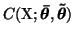with respect to the posterior means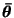and variances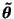of the unknown variables. As usual, however, it makes sense utilising the particular structure of the function to be minimised.

Those parameters which are means or log-std of Gaussian distributions, e.g., mb, mvB, va and vvx, can be solved in the same way as the parameters of Gaussian distribution where solved in Sect. 6.1. Since the parameters have Gaussian priors, the equations do not have analytical solutions, but Newton-iteration can be used. For each Gaussian, the posterior mean and variance of the parameter governing the mean is solved first by assuming all other variables constant and then the same thing is done for the log-std parameter, again assuming all other variables constant.

Since the mean and variance of the output of the network and thus also the cost function was computed layer by layer, it is possible to use the ordinary back-propagation algorithm to evaluate the partial derivatives of the part Cp of the cost function w.r.t. the posterior means and variances of the sources, weights and biases. Assuming the derivatives computed, let us first take a look at the posterior variances.

The effect of the posterior variancesof sources, weights and biases on the part Cp of the cost function is mostly due to the effect on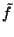which is usually very close to linear (this was also the approximation made in the evaluation of the cost function). The termshave a linear effect on the cost function, as is seen in (21), which means that the over all effect of the termson Cp is close to linear. The partial derivative of Cp with respect tois therefore roughly constant and it is reasonable to use the following fixed point equation to update the variances: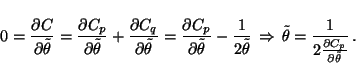(27)

The remaining parameters to be updated are the posterior means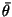of the sources, weights and biases. For those parameters it is possible to use Newton iteration since the corresponding posterior variancesactually contain the information about the second order derivatives of the cost function C w.r.t.. It holds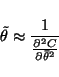(28)

and thus the step in Newton iteration can be approximated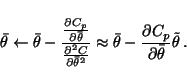(29)

Equation (29) would be exact if the posterior pdf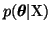were exactly Gaussian. This would be true if the mapping f were linear. The approximation in (29) is therefore good as long as the function f is roughly linear around the current estimate of.Next: Avoiding Problems Originating from Up: Nonlinear Factor Analysis Previous: Cost Function
Harri Lappalainen
2000-03-03